# Isolated Uniform-Density Sphere

Here we derive the interior structural properties of an isolated uniform-density sphere using all three solution strategies. While deriving essentially the same solution three different ways might seem like a bit of overkill, this approach proves to be instructive because (a) it forces us to examine the structural behavior of a number of different physical parameters, and (b) it illustrates how to work through the different solution strategies for one model whose structure can in fact be derived analytically using any of the techniques. As we shall see when studying other self-gravitating configurations, the three strategies are not always equally fruitful.

## Solution Technique 1

Adopting solution technique #1, we need to solve the integro-differential equation,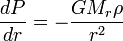$~\frac{dP}{dr} = - \frac{GM_r \rho}{r^2}$

appreciating that,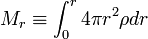$M_r \equiv \int_0^r 4\pi r^2 \rho dr$ .

For a uniform-density configuration,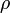$~\rho$ =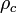$~\rho_c$ = constant, so the density can be pulled outside the mass integral and the integral can be completed immediately to give,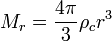$M_r = \frac{4\pi}{3}\rho_c r^3$ .

Hence, the differential equation describing hydrostatic balance becomes,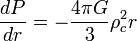$\frac{dP}{dr} = - \frac{4\pi G}{3} \rho_c^2 r$ .

Integrating this from the center of the configuration — where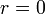$~r=0$ and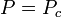$~P = P_c$ — out to an arbitrary radius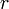$~r$ that is still inside the configuration, we obtain,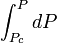$~ \int_{P_c}^P dP$$~=$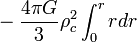$~- \frac{4\pi G}{3} \rho_c^2 \int_0^r r dr$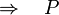$~ \Rightarrow ~~~ P$$~=$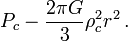$~P_c - \frac{2\pi G}{3} \rho_c^2 r^2 \, .$

We expect the pressure to drop to zero at the surface of our spherical configuration — that is, at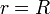$~r=R$ — so the central pressure must be,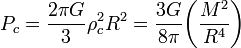$P_c = \frac{2\pi G}{3} \rho_c^2 R^2 = \frac{3G}{8\pi}\biggl( \frac{M^2}{R^4} \biggr)$ ,

where$~M$ is the total mass of the configuration. Finally, then, we have,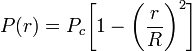$P(r) = P_c\biggl[1 - \biggl(\frac{r}{R}\biggr)^2 \biggr]$ .

## Solution Technique 3

Adopting solution technique #3, we need to solve the algebraic expression,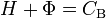$~H + \Phi = C_\mathrm{B}$ .

in conjunction with the Poisson equation,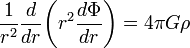$\frac{1}{r^2} \frac{d }{dr} \biggl( r^2 \frac{d \Phi}{dr} \biggr) = 4\pi G \rho$ .

Appreciating that, as shown above, for a uniform density ($~\rho$ = ρc = constant) configuration,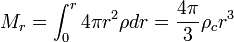$M_r = \int_0^r 4\pi r^2 \rho dr = \frac{4\pi}{3}\rho_c r^3$ ,

we can integrate the Poisson equation once to give,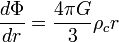$\frac{d\Phi}{dr} = \frac{4\pi G}{3} \rho_c r$ ,

everywhere inside the configuration. Integrating this expression from any point inside the configuration to the surface, we find that,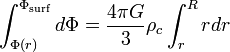$\int_{\Phi(r)}^{\Phi_\mathrm{surf}} d\Phi = \frac{4\pi G}{3} \rho_c \int_r^R r dr$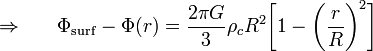$\Rightarrow ~~~~~ \Phi_\mathrm{surf} - \Phi(r) = \frac{2\pi G}{3} \rho_c R^2 \biggl[ 1- \biggl(\frac{r}{R} \biggr)^2 \biggr]$

Turning to the above algebraic condition, we will adopt the convention that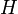$~H$ is set to zero at the surface of a barotropic configuration, in which case the constant, CB, must be,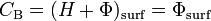$~C_\mathrm{B} = (H + \Phi)_\mathrm{surf} = \Phi_\mathrm{surf}$ .

Therefore, everywhere inside the configuration$~H$ must be given by the expression,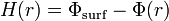$~H(r) = \Phi_\mathrm{surf} - \Phi(r)$ .

Matching this with our solution of the Poisson equation, we conclude that, throughout the configuration,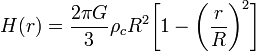$H(r) = \frac{2\pi G}{3} \rho_c R^2 \biggl[ 1- \biggl(\frac{r}{R} \biggr)^2 \biggr]$ .

Comparing this result with the result we obtained using solution technique #1, it is clear that throughout a uniform-density, self-gravitating sphere,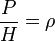$\frac{P}{H} = \rho$ .

## Solution Technique 2

Adopting solution technique #2, we need to solve the following single, 2nd-order ODE: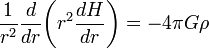$\frac{1}{r^2} \frac{d }{dr} \biggl( r^2 \frac{d H}{dr} \biggr) = - 4\pi G \rho$ .

Appreciating again that, for a uniform density ($~\rho$ = ρc = constant) configuration,$M_r = \int_0^r 4\pi r^2 \rho dr = \frac{4\pi}{3}\rho_c r^3$ ,

we can integrate the 2nd-order ODE once to give,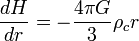$\frac{dH}{dr} = -\frac{4\pi G}{3} \rho_c r$ ,

everywhere inside the configuration. Integrating this expression from any point inside the configuration to the surface — where, again, we adopt the convention that$~H$ = 0 — we find that,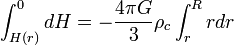$\int_{H(r)}^{0} dH = - \frac{4\pi G}{3} \rho_c \int_r^R r dr$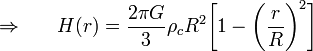$\Rightarrow ~~~~~ H(r) = \frac{2\pi G}{3} \rho_c R^2 \biggl[ 1- \biggl(\frac{r}{R} \biggr)^2 \biggr]$ .

## Summary

From the above derivations, we can describe the properties of a uniform-density, self-gravitating sphere as follows:

• Mass:
Given the density, ρc, and the radius, R, of the configuration, the total mass is,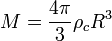$M = \frac{4\pi}{3} \rho_c R^3$ ;

and, expressed as a function of M, the mass that lies interior to radius r is,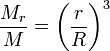$\frac{M_r}{M} = \biggl(\frac{r}{R} \biggr)^3$ .

• Pressure:
Given values for the pair of model parameters c,R), or (M,R), or c,M), the central pressure of the configuration is,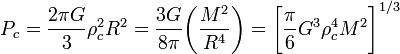$P_c = \frac{2\pi G}{3} \rho_c^2 R^2 = \frac{3G}{8\pi}\biggl( \frac{M^2}{R^4} \biggr) = \biggl[ \frac{\pi}{6} G^3 \rho_c^4 M^2 \biggr]^{1/3}$ ;

and, expressed in terms of the central pressure Pc, the variation with radius of the pressure is,$P(r) = P_c \biggl[ 1 -\biggl(\frac{r}{R} \biggr)^2 \biggr]$ .

• Enthalpy:
Throughout the configuration, the enthalpy is given by the relation,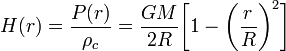$H(r) = \frac{P(r)}{ \rho_c} = \frac{GM}{2R} \biggl[ 1 -\biggl(\frac{r}{R} \biggr)^2 \biggr]$ .

• Gravitational potential:
Throughout the configuration — that is, for all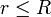$r \leq R$ — the gravitational potential is given by the relation,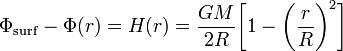$\Phi_\mathrm{surf} - \Phi(r) = H(r) = \frac{G M}{2R} \biggl[ 1- \biggl(\frac{r}{R} \biggr)^2 \biggr]$ .

Outside of this spherical configuration— that is, for all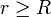$r \geq R$ — the potential should behave like a point mass potential, that is,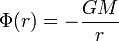$\Phi(r) = - \frac{GM}{r}$ .

Matching these two expressions at the surface of the configuration, that is, setting Φsurf = − GM / R, we have what is generally considered the properly normalized prescription for the gravitational potential inside a uniform-density, spherically symmetric configuration: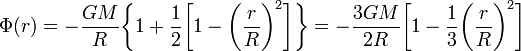$\Phi(r) = - \frac{G M}{R} \biggl\{ 1 + \frac{1}{2}\biggl[ 1- \biggl(\frac{r}{R} \biggr)^2 \biggr] \biggr\} = - \frac{3G M}{2R} \biggl[ 1 - \frac{1}{3} \biggl(\frac{r}{R} \biggr)^2 \biggr]$ .

We see that, for a given value of ρc, the relationship between the configuration's total mass and radius is,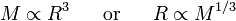$M \propto R^3 ~~~~~\mathrm{or}~~~~~R \propto M^{1/3}$ .

• Central- to Mean-Density Ratio:
Because this is a uniform-density structure, the ratio of its central density to its mean density is unity, that is,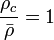$\frac{\rho_c}{\bar{\rho}} = 1$ .

# Uniform-Density Sphere Embedded in an External Medium

For the isolated uniform-density sphere, discussed above, the surface of the configuration was identified as the radial location where the pressure drops to zero. Here we embed the sphere in a hot, tenuous medium that exerts a confining "external" pressure,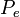$~P_e$, and ask how the configuration's equilibrium radius,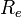$~R_e$, changes in response to this applied external pressure, for a given (fixed) total mass and central pressure,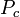$~P_c$.

Following solution technique #1, the derivation remains the same up through the integration of the hydrostatic balance equation to obtain the relation,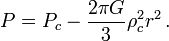$P = P_c - \frac{2\pi G}{3} \rho_c^2 r^2 \, .$

Now we set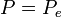$~P = P_e$ at the surface of our spherical configuration — that is, at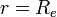$~r=R_e$ — so we can write,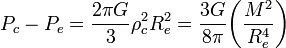$P_c - P_e = \frac{2\pi G}{3} \rho_c^2 R_e^2 = \frac{3G}{8\pi}\biggl( \frac{M^2}{R_e^4} \biggr)$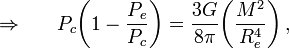$\Rightarrow ~~~~~ P_c \biggl( 1 - \frac{P_e}{P_c} \biggr) = \frac{3G}{8\pi}\biggl( \frac{M^2}{R_e^4} \biggr) \, ,$

where M is the total mass of the configuration. Solving for the equilibrium radius, we have,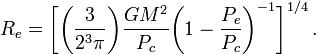$R_e = \biggl[ \biggl( \frac{3}{2^3\pi} \biggr) \frac{G M^2}{P_c} \biggl( 1 - \frac{P_e}{P_c} \biggr)^{-1} \biggr]^{1/4} \, .$

As it should, when the ratio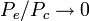$~P_e/P_c \rightarrow 0$, this relation reduces to the one obtained, above, for the isolated uniform-density sphere, namely,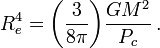$R_e^4 = \biggl( \frac{3}{8\pi} \biggr) \frac{G M^2}{P_c} \, .$

 © 2014 - 2020 by Joel E. Tohline |   H_Book Home   |   YouTube   | Appendices: | Equations | Variables | References | Ramblings | Images | myphys.lsu | ADS |